Vibroengineering PROCEDIA

Published: 07 May 2021

# Dynamic safety measurement-control technology for intelligent connected vehicles based on digital twin system

Xingbin Chen1
Xinhe Min2
Nini Li3
Wei Cao4
Shunren Xiao5
Guanting Du6
Peng Zhang7
1, 2, 3, 4, 6, 7Guangzhou Mechanical Engineering Research Institute Co., Ltd., Guangzhou, 510700, China
5, 1, 7School of Mechanical and Automotive engineering, South China University of Technology, Guangzhou, 510641, China
2, 3, 4, 6, 1, 7CRAT Testing and Certification Company Ltd., Guangzhou, 510700, China
Corresponding Author:
Xingbin Chen
Corresponding Author:
Peng Zhang
Views 267

#### Abstract

As the number of connected vehicles increases, the intelligence levels become more and more uneven, so the problem how to determine the dynamic safety of autonomous driving behavior in the mixed-flow traffic system is significantly increased. To solve many difficult problems related to human-vehicle-road perception, decision, control coordination, and reliability evaluation in the intelligent networked scenario, this study establishes a dynamic Game model with multi-source information in the intelligent networked environment, to carry out the measurements and control evaluations for autonomous vehicle dynamics models, forward and backward active safety control, and mixed traffic trajectory optimization planning based on the optimal solution strategy. A digital twin test verification platform with semi-physical environment and hardware-in-the-loop (HIL) simulation to control the accuracy for making dynamic safety decision for the intelligent networked vehicle is developed by combining with the V2X real intelligent transportation system and smart laboratory virtual simulation test technology, which realizes the complex and dynamic safety decision goals for autonomous vehicle in different connected levels of the mixed-flow traffic environments, such as multi-agent perception, multi-source information transmission, vehicle control, vehicle-to-vehicle communication, and vehicle-to-road coordination. The study is conducive to improving the test efficiency and index evaluation integrity of the intelligent networked system, reducing the test costs, proving the behaviors of Game interaction and stress safety response under vehicle-to-road environment, enhancing the robustness and applicability of automatic driving technology, and improving the traffic efficiency and safety of mixed-flow traffic in the intelligent network.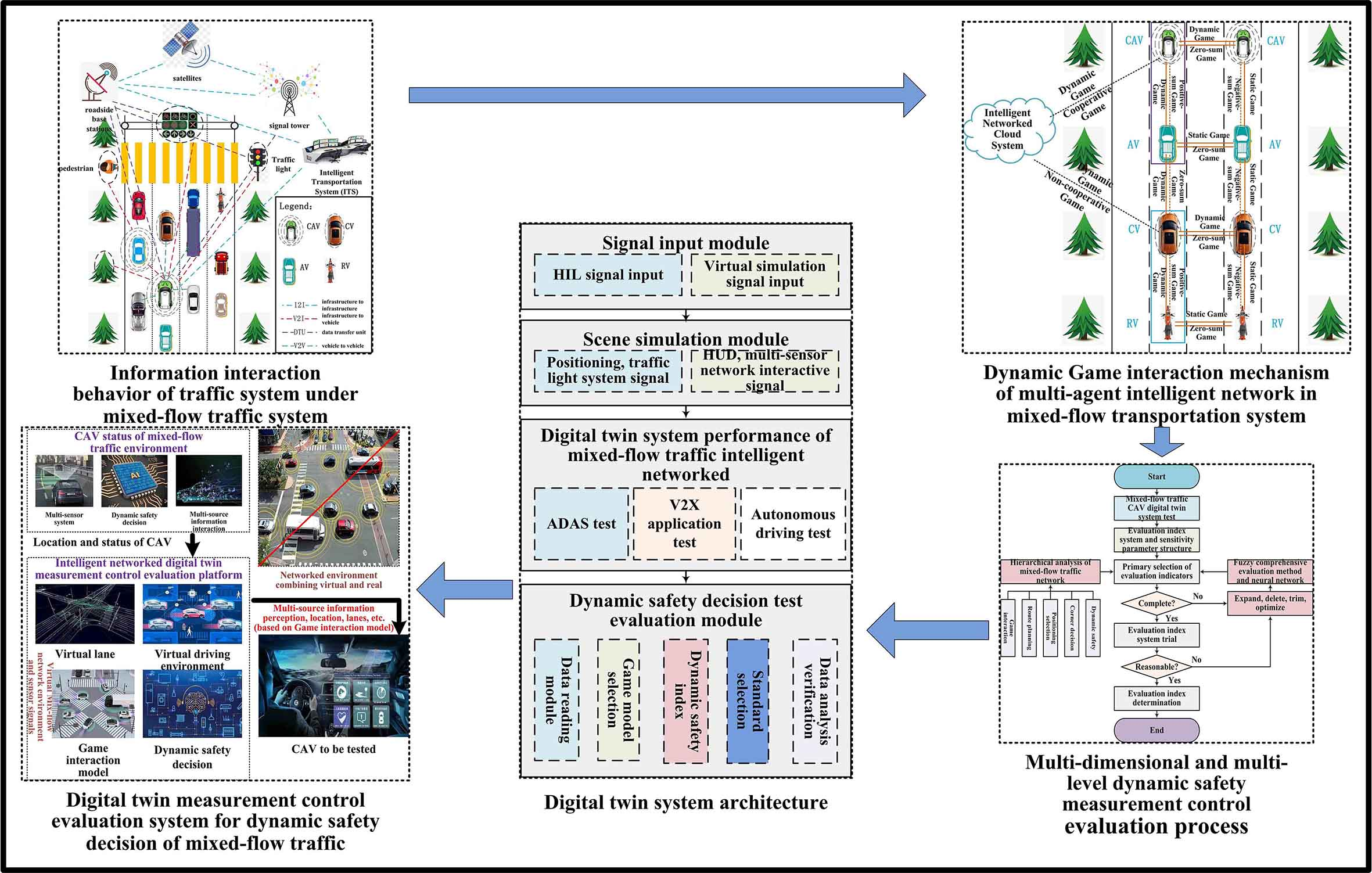#### Highlights

• Game Interactive Behavior Modeling for Dynamic Safety Decision based on surrounding heterogeneous vehicles, traffic environment, objects and even people at different grade intelligence.
• Hierarchical collaboration dynamic in vehicle-road perception, decision, and control are conducted for homogeneous or heterogeneous agents aiming at networked environment.
• Digital twin measurement control evaluation system is studied based on virtual-real combination of intelligent network to set up a multi-dimensional and multi-level dynamic safety evaluation index system for determining the weights of evaluation aspects, elements, factors and indexes at all levels by analytic hierarchy process (AHP).
• Digital twin test and verification platform development for dynamic safety decision and control accuracy with optimized semi-physical environment.

## 2. Game interactive behavior modeling for dynamic safety decision

Due to the complexity of mixed-flow traffic scenes, the connected vehicles when driving autonomously will be affected by surrounding heterogeneous vehicles, traffic environment, objects and even people at different grade intelligence. When carrying out driving behaviors such as path selection or positioning control, the vehicle will inevitably generate Game interaction with different subjects in the mixed-flow system.

In the V2X context, the information communication and sharing takes place between the connected autonomous vehicles and surrounding vehicles, and also cooperative and competitive relationships occur between any two adjacent vehicles. The Game theory can be used to characterize the uncertain strategy of the mixed-flow traffic system, optimize the path selection or positioning control, and then predict the driving behavior to realize the dynamic real-time information interaction between vehicles, or vehicles-roads in all directions.

The intelligent networked cloud can generate Game interaction relationship with CAVs in the mixed-flow traffic, and it is a dynamic Game with multiple reciprocations. It is easy to form a cooperative relationship with CAVs with automatic driving function, thus forming cooperative Game, while it is easy to form non-cooperative Game with CVs due to the selfish behavior of some drivers. The Game phenomenon in the mixed-flow traffic is more complicated, without external intervention, the Game interactions between vehicles are almost non-cooperative. However, from the perspective of the intelligent networked cloud, it is necessary to encourage cooperation between vehicles with different connectivity degrees in the mixed-flow traffic, and realize the “intelligence” for non-networked vehicles through imitating-following connected vehicles, thus, the static Games and non-cooperative Games in the mixed-flow traffic are transformed into dynamic Games and cooperative Games. Furthermore, the intelligent networked environment system is realized as the optimized option, as shown in Fig. 2.

The driving process of regional vehicles can be regarded as a dynamic Game process. The CAV makes safety decisions to pierce safely into the traffic by keeping a proper distance between the front and rear vehicles, which can be regarded as a dynamic repeated Game process with limited times and multiple stages. In the mixed-flow traffic with intelligent network environment, the Game process of the remaining traffic agents can be regarded as N-source information under the specific road grade, lane alignment, driving route and meteorological conditions.

(1) Vehicle agent collection:

1

where, $n$ is the total number of vehicles, $\omega$ is the proportion of different type vehicles.

Fig. 1Information interaction behavior of traffic system under mixed-flow traffic system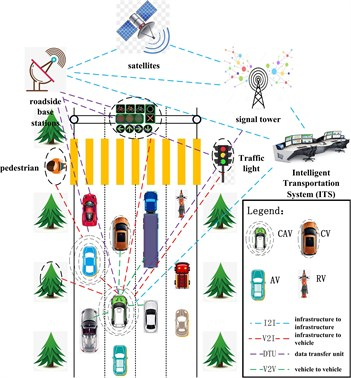Fig. 2Dynamic Game interaction mechanism of multi-agent intelligent network in mixed-flow transportation system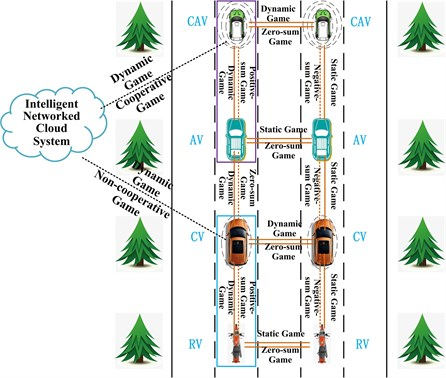(2) Game collection of a single vehicle $i$:

2

(3) Corresponding Game decision probability:

3
$\left\{\begin{array}{l}{X}_{{i}_{cv}}=\left\{{x}_{1}^{\left({i}_{cv}\right)},{x}_{2}^{\left({i}_{cv}\right)},\cdots ,{x}_{{m}_{cv}}^{\left({i}_{cv}\right)}\right\},\\ {X}_{{i}_{cav}}=\left\{{x}_{1}^{\left({i}_{cav}\right)},{x}_{2}^{\left({i}_{cav}\right)},\cdots ,{x}_{{m}_{cav}}^{\left({i}_{cav}\right)}\right\},\\ {X}_{{i}_{av}}=\left\{{x}_{1}^{\left({i}_{av}\right)},{x}_{2}^{\left({i}_{av}\right)},\cdots ,{x}_{{m}_{av}}^{\left({i}_{av}\right)}\right\},\\ {X}_{{i}_{rv}}=\left\{{x}_{1}^{\left({i}_{rv}\right)},{x}_{2}^{\left({i}_{rv}\right)},\cdots ,{x}_{{m}_{rv}}^{\left({i}_{rv}\right)}\right\}.\end{array}\right\$

In Eq. (3), ${x}_{k}^{i}\ge 0$, $k=1,2,\cdots ,{m}_{i}$, $\sum _{k=1}^{{m}_{i}}{x}_{k}^{i}=1$.

(4) Mixed Game strategy. ${x}^{\left(i\right)}$ represents a vehicle $i$ choosing a certain strategy from the Game collection, and the probability in a pure Game strategy ${s}_{k}^{\left(i\right)}$ is ${x}_{k}^{\left(i\right)}$. In the mixed-flow system, the vehicle $i$ replaces ${x}^{\left(i\right)}$ with another dynamic mixed Game strategy, while keeping the other strategies unchanged:

4
$x‖{z}^{\left(i\right)}=\left({x}^{\left(1\right)},\cdots ,{x}^{\left(i-1\right)},{z}^{\left(i\right)},{x}^{\left(i+1\right)},\cdots ,{x}^{\left(n\right)}\right).$

(5) Single type Game effect. When a certain Game strategy is selected, a collection trend $s=\left({s}^{\left(1\right)},{s}^{\left(2\right)},\cdots ,{s}^{\left(n\right)}\right)$ of the Game is formed, where, ${s}^{\left(i\right)}\in {s}_{i}$, for each trend $s$, the Game effect obtained by each different type vehicle is:

5
$\left\{\begin{array}{l}{P}_{cv}^{i}={P}_{{i}_{cv}}\left(s\right),\\ {P}_{cav}^{i}={P}_{{i}_{cav}}\left(s\right),\\ {P}_{av}^{i}={P}_{{i}_{av}}\left(s\right),\\ {P}_{rv}^{i}={P}_{{i}_{rv}}\left(s\right).\end{array}\right\$

(6) Expectation effect of mixed-flow Games. The expected effect of each different type vehicle $i$ in the mixed-flow strategy trend $x=\left({x}^{\left(1\right)},{x}^{\left(2\right)},\cdots ,{x}^{\left(n\right)}\right)$ is:

6
$\left\{\begin{array}{l}{E}_{cv}^{i}={E}_{{i}_{cv}}\left(x\right),\\ {E}_{cav}^{i}={E}_{{i}_{cav}}\left(x\right),\\ {E}_{av}^{i}={E}_{{i}_{av}}\left(x\right),\\ {E}_{rv}^{i}={E}_{{i}_{rv}}\left(x\right).\end{array}\right\$

(7) Multi-agent Game of mixed-flow traffic in the intelligent network environment:

7
$G=\left[I,\left\{{X}_{i}\right\},\left\{{P}_{i}\right\}\right].$

## 3. Influencing factors of vehicle dynamic safety decision under Game behavior

Different from most studies for location recognition and trajectory planning of autonomous vehicles with single “real-time motion interaction”, this paper covers hierarchical collaboration dynamic study for vehicle-road perception, decision, and control of homogeneous or heterogeneous agents.

(1) Collision potential. The Game interaction process of multi-agent in the mixed traffic can use time-to-collision (TTC) between vehicles to evaluate the driving safety. Assuming that the relative distance between adjacent vehicles at any time $t$ is ${L}_{r}$:

8
${L}_{r}\left(t\right)={L}_{r}\left({t}_{0}\right)+\left[{v}_{1,f}\left({t}_{0}\right)-{v}_{1,b}\left({t}_{0}\right)\right]\left(t-{t}_{0}\right)+{\int }_{{t}_{0}}^{t}{\int }_{t}^{\tau }\left[{a}_{1,f}\left(l\right)-{a}_{1,b}\left(l\right)\right]dsd\tau .$

In Eq. (8), ${v}_{1,f}\left({t}_{0}\right)$, ${v}_{1,b}\left({t}_{0}\right)$ are respectively the speeds of two adjacent vehicles in front and behind (m/s). ${a}_{1,f}\left(l\right)$, ${a}_{1,b}\left(l\right)$ are the adjacent acceleration.

Then the collision time is:

9
$TTC=t-{t}_{0}=\frac{-∆v+\sqrt{{\left(∆v\right)}^{2}+4∆a∆L}}{2∆a}.$

In Eq. (9), the relative speed difference is $∆v={v}_{1,f}\left({t}_{0}\right)-{v}_{1,b}\left({t}_{0}\right)$, and $∆v>0$ is required. The relative acceleration difference is $∆a={a}_{\mathrm{m}\mathrm{a}\mathrm{x}}-{a}_{1,f\mathrm{m}\mathrm{a}\mathrm{x}}$, where, the maximum braking deceleration of the decision vehicle agent to avoid rear-end collision is ${a}_{\mathrm{m}\mathrm{a}\mathrm{x}}$, and the maximum deceleration of adjacent vehicle at a certain moment is ${a}_{1,f\mathrm{m}\mathrm{a}\mathrm{x}}$. The relative safety distance is $∆L={L}_{r}\left({t}_{0}\right)$.

(2) Relative driving space and relative speed. The driving space $∆{L}_{i}$ in front of the vehicle (including the front space distance and length of vehicle) and relative speed $∆{v}_{i}$ are important influencing factors for the driving intention of the vehicle agent’s lane selection. In order to better qualitatively analyze the relationship between $∆{L}_{i}$ and $∆{v}_{i}$, a membership of fuzzy set is introduced as follows:

10
$f\left(x,\sigma ,∆L\right)=e\frac{{\left(x-∆L\right)}^{2}}{2{\sigma }^{2}}.$

(3) Planning driving time decision under safe distance. According to the factors such as the boundary value of the collision risk safety degree, adjustment margin of safety guarantee, TTC experience threshold, the driving time of CAV within the limited safe distance is obtained as:

11
${t}^{dr}=\frac{-∆{v}_{i\text{'},i}+\sqrt{{\left(∆{v}_{i\text{'},i}\right)}^{2}+4∆{a}_{i\text{'},i}∆{L}_{i\text{'},i}}}{2∆{a}_{i\text{'},i}}.$

In Eq. (11), $∆{L}_{i\text{'},i}$, $∆{v}_{i\text{'},i}$, $∆{a}_{i\text{'},i}$ are respectively the relative displacement, relative speed and relative acceleration of vehicle agent from a certain position to the target safe position selected by the Game.

## 4. Study on digital twin measurement control evaluation system based on virtual-real combination of intelligent network

The digital twin measurement control evaluation method based on the virtual real combination is helpful to solve these problems, such as real-time calculation performance of HIL test and accurate performance of simplified system, which can save a lot of time, and have high repeatability under the same test conditions.

Based on the multi-dimensional and multi-level dynamic safety evaluation index system of human-vehicle-traffic system, the weights of evaluation aspects, elements, factors and indexes at all levels are determined by the analytic hierarchy process (AHP). The multi-dimensional and multi-level dynamic safety evaluations are studied through the methods of fuzzy comprehensive evaluation and neural network evaluation, as well as the overall evaluation for the passing safety of mixed-flow traffic system, as shown in Fig. 3.

(1) Kinematic and geometric characteristics of networked vehicles. They include collision time, maximum longitudinal acceleration/deceleration, maximum lateral acceleration, braking time, lane post-invasion time, instantaneous collision probability and safety limits, etc.

(2) Mixed-flow traffic environment characteristics. They mainly include road conditions, traffic facilities, landforms, meteorological conditions, and traffic activities of other participants.

(3) Dynamic safety features. The evaluations are mainly from two aspects: driving safety and information safety.

1) Driving safety. Driving safety program mainly evaluates the interactive behavior safety of CAV with surrounding traffic participants (other vehicles, pedestrians, bicycles, etc.) during autonomous driving operations, as well as the safety achieves avoiding and minimizing collision risks when the CAV encounters a collision hazard or anticipates a collision.

2) Information safety. Information safety program conducts corresponding evaluations on protective countermeasures due to information transmission hazards (such as security vulnerabilities), communication network delays or jams, safety threats, and malicious intrusions in the information interaction process between CAVs and outside world.

Generally, the trajectory measurement control evaluations of CAVs are based on complex numerical simulation analysis by taking the whole system as the object, which leads to a lack of stability and engineering operability for dynamic trajectory measurement and control. With focus on establishing a dynamic safety decision model, a test evaluation method is proposed for forward and backward active safety control or mixed-flow traffic trajectory optimization planning based on the optimal solution strategy, the internal correlation between the dynamic safety and traffic stability is revealed through the network hierarchy and multi-source information agent classification, so that the influence law of each sensitivity parameter is explored.

## 5. Development of digital twin measurement control evaluation platform

Focusing on the problems of dynamic safety decision and trajectory positioning for the Game measurement control of intelligent networked vehicles, it is proposed to conduct a deeper study on the multi-source information identification and Game safety decision with heterogeneous mixed-flow traffic characteristics. Then a digital twin measurement control evaluation platform based on the virtual and real combination of intelligent network is constructed to evaluate the effect of dynamic Game safety decision.

Fig. 3Multi-dimensional and multi-level dynamic safety measurement control evaluation process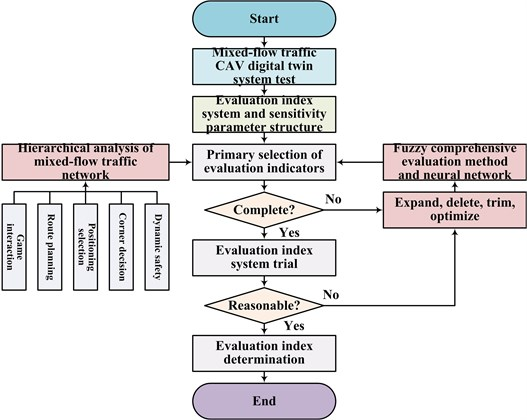Fig. 4Digital twin system architecture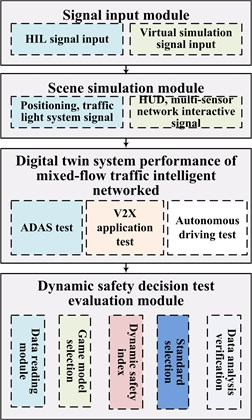(1) Signal input module. The digital twin test system receives the scene information and signal type required by the test device from the HIL and virtual simulation module, which generates the required physical-level signal or true value signal, and input to the digital twin system interface, such as image, RCS echo, LTE-V signals, homogenous or heterogeneous networked vehicle participants in mixed-flow traffic, multi-source information lists.

(2) Scene simulation module.

1) The simulation scene is constructed, and simulated with vehicle position, speed, driving path and positioning selection can be planned globally. 2) Controller sends simulated vehicle information.3) Positioning device is used to determine the current position of vehicle. 4) The signal input device is dependent on the scene, which parameters are dynamically adjusted. 5) Data transmission network. 6) HIL signals support multi-terminal access, and the data can be uploaded through LTE-V/4G/5G networks. 7) A connection is established between the test platform and local test system.

(3) Digital twin system test performance. It can conduct multi-dimensional and multi-level evaluation tests of dynamic safety for the system Game interaction, route planning, positioning selection, lane change decision and other aspects, as well as the overall test of traffic safety in mixed-flow traffic system.

(4) Dynamic safety decision testing and evaluation module. Vehicle information and warning messages uploaded by the local test system, receive simulated vehicle information, and synchronize with system data source, so as to carry out lane Game interactive simulation and trajectory positioning control. It is needed to evaluate the test results of safety distance, relative speed, collision time, etc.

Based on the V2X real intelligent transportation system data, the actual test results are extracted through virtual hardware and virtual sensors. In addition, the intelligent networked virtual simulation technology is integrated, which can set up intelligent networked scenes for the multi-source subjects and information in the complex mixed-flow traffic system. So, the driving status of various levels CVs or heterogeneous vehicles, the trajectory positioning decision are adjusted and optimized through the forward and backward response to obtain a more stable and reliable control strategy.

## 6. Conclusions

Focused on the safety driving decision accuracy problems in mixed-flow traffic system, this paper studies a multi-source dynamic Game interaction model, a multi-agent dynamic Game simulation test platform is established under the intelligent networked scene, to test and evaluate the dynamic safety decision control accuracy for autonomous vehicles, thus, a scientific and reasonable evaluation system for intelligent networked dynamic safety standards is established. The results can significantly improve the safety and reliability of connected vehicles, and reduce the delay rate of feedback control response and the error rate of trajectory perception planning.

1) Game interaction relation Construction for heterogeneous mixed-flow traffic

With the goals of dynamic safety decision for autonomous vehicles, the driving path planning can be realized in advance through “Game mutual identification” for homogeneous networked vehicles, the driving trajectory optimization can be realized through “real-time motion interaction” and “Game feedback” between trajectory representations for heterogeneous non-networked vehicles, however, the driving intention can be realized through the “multi-source information collection” and “Game decision” between the safety parameters adjustment decisions for heterogeneous non-networked multi-environment-agent.

2) Digital twin test and verification platform development for dynamic safety decision and control accuracy with optimized semi-physical environment

A test verification method is proposed taking into account the on-site measurement control and virtual simulation evaluation, and a related software and hardware platform is developed, with integrated modules such as network and non-network compatible driving environment, complex mixed-flow traffic system, multi-source information identification and sensing system, autonomous vehicle dynamics, test controller, driving trajectory planning and deviation compensation, components and vehicle dynamic safety decision evaluation modules. The dynamic safety decision and trajectory location control strategy of the intelligent networked vehicle are studied from the perspective of safety and reliability, so as to analyze the trajectory location characteristics of the autonomous vehicle under the complex mixed-flow traffic system with multi-source information and Game interaction between networked and non-networked agents. The dynamic safety decision accuracy is verified through the automatic driving digital twin test technology, the generation mechanism of trajectory positioning deviation and influence law of deviation source sensitivity parameters are summarized, in order to form a multilevel hierarchy deviation compensation mechanism according to the classification compensation method of each deviation source.

3) Study on theories and methods of dynamic safety decision and trajectory positioning reliability evaluation for intelligent networked vehicles

Combined with key technical characterization parameters such as driving reliability, driving control reliability and position recognition reliability, the corresponding evaluation theory was proposed, the intelligent networked digital twin evaluation test platform was improved and perfected, and the trajectory safety reliability testing standard was established. Thus, the evaluation method proved the traceability and verifiability.

#### Acknowledgements

This work was supported by the National Key Research and Development Plan of China (Grant No. 2017YFB1301400), the Guangdong Provincial Enterprises Key Laboratory Foundation about Mid-High-End Industrial Robot Technology (Grant No. 2018B030323027), and the Postdoctoral project of Guangzhou Academy of Mechanical Sciences Co., Ltd. (Grant No. 1014300036).

#### Cited by

SecCDV: A Security Reference Architecture for Cybertwin-Driven 6G V2X